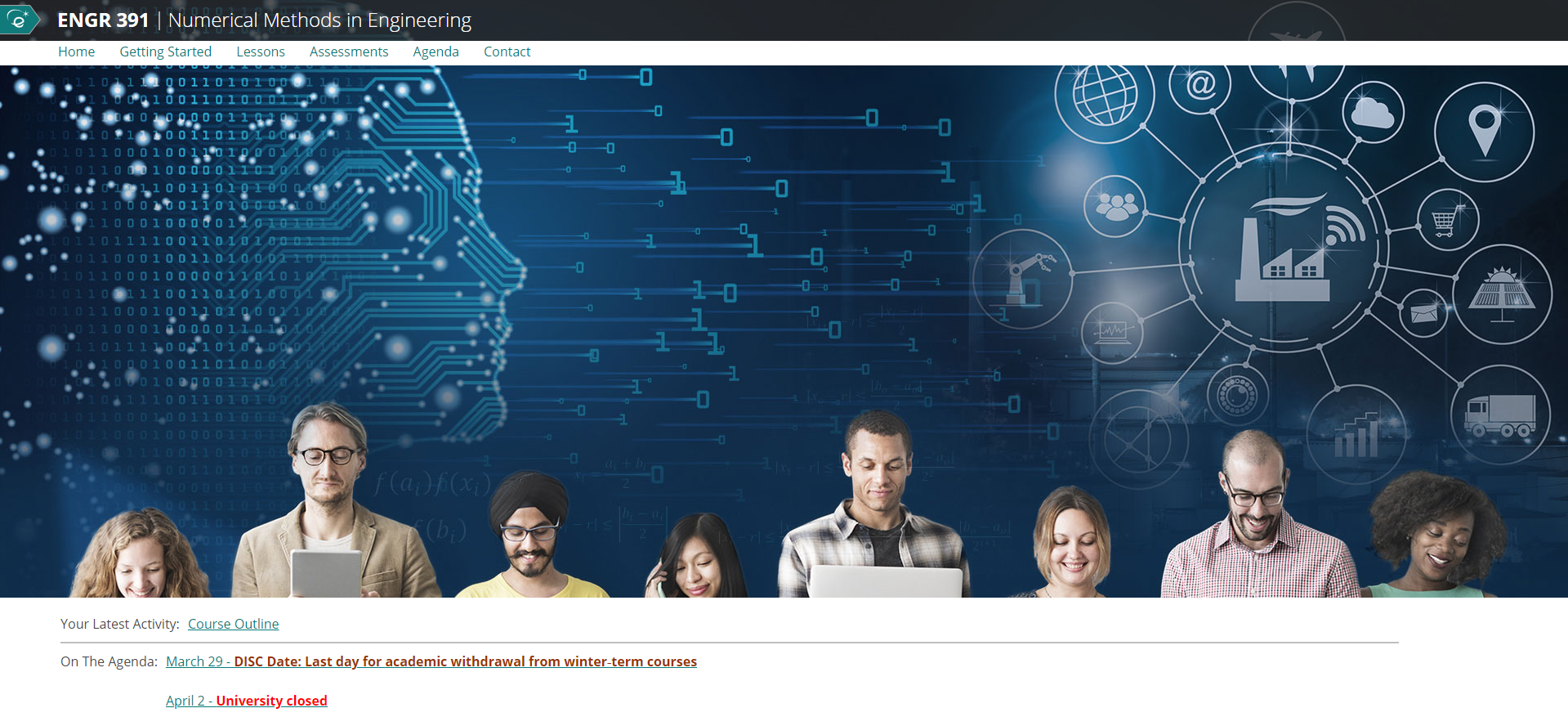### Project Description

This course aims to teach main numerical methods used in various engineering fields, their limitations, and how they can be implemented using a data analysis tool.

Topics covered in this course: Roots of algebraic and transcendental equations; function approximation; numerical differentiation; numerical integration; solution of simultaneous algebraic equations; numerical integration of ordinary differential equations.